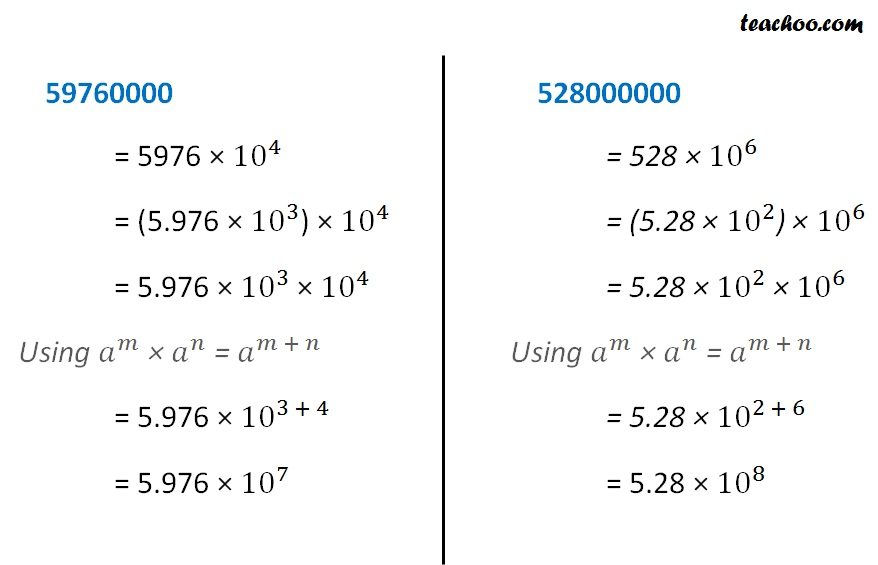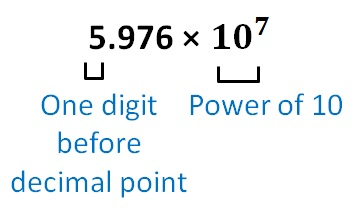Standard Form

Chapter 13 Class 7 Exponents and Powers
Concept wise

Suppose we have two numbers

59760000 & 528000000

How do we compare them?

Comparing them becomes difficult when the number are large.

So, we write both number in standard formSo, our two numbers are

5.976 × 10 7

5.28 × 10 8

Higher Power

So,

5.28 × 10 8 > 5.976 × 10 7

Here,

Standard form isSo, in standard form

• We write number as power of 10
• There is only 1 digit before decimal point

Let’s take some more examples

28488

= 2.8488 × 10 4

### 8680 in standard form

8680 = 868 × 10 1

= 8.68 × 10 2 × 10 1

Usinga m × a n = a m + n

= 8.68 × 10 2 + 1

= 8.68 × 10 3

### 92058 in standard form

92058

= 9.2058 × 10 4

### 35.89 in standard form

35.89

= 3589/100

= 3589 × 1/100

= 3589 × 1/10 2

= (3.589 × 10 3 ) × 1/10 2

= 3.589 × 10 3 /10 2

= 3.589 × 10 3 - 2

= 3.589 × 10 1      (Using  a m /a n  =  a m - n )

### Write 2.008 in standard form

2.008

= 2.008 × 10 0

0.00008

= 8/100000

= 8/10 5

= 8 × 10 -5

### Write 0.0092 in standard form

0.0092

= 92/10000

= 92 × 1/10000

= 9.2 × 10 × 1/10000

= 9.2 × 1/1000

= 9.2 × 1/10 3

= 9.2 × 10 -3

Get live Maths 1-on-1 Classs - Class 6 to 12

### Transcript

59760000 = 5976 × 〖10〗^4 = (5.976 × 〖10〗^3) × 〖10〗^4 = 5.976 × 〖10〗^3 × 〖10〗^4 Using 𝑎^𝑚 × 𝑎^𝑛 = 𝑎^(𝑚 + 𝑛) = 5.976 × 〖10〗^(3 + 4) = 5.976 × 〖10〗^7 528000000 = 528 × 〖10〗^6 = (5.28 × 〖10〗^2) × 〖10〗^6 = 5.28 × 〖10〗^2 × 〖10〗^6 Using 𝑎^𝑚 × 𝑎^𝑛 = 𝑎^(𝑚 + 𝑛) = 5.28 × 〖10〗^(2 + 6) = 5.28 × 〖10〗^8